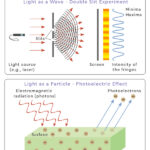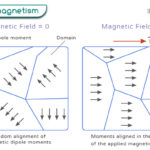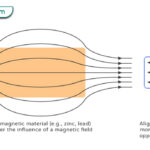Home / Physics / Elastic Potential Energy

# Elastic Potential Energy

Elastic potential energy, also known as elastic energy, is the energy stored in an elastic object when a force is applied to deform it. The energy is stored as long as the force is present. When the force is released, the energy is converted into another form according to the conservation of energy law. The applied force must be within a specific limit, known as the elastic limit.

## Examples

The following objects from everyday life store elastic potential energy:

• The spring of a wind-up clock
• The stretched bow of an archer
• A bent diving board just before a diver takes a dive
• The rubber band of a stretched slingshot
• When a bouncy ball hits the wall or floor

## How to Calculate Elastic Potential Energy

According to Hooke’s law, the magnitude of the elastic force is proportional to the length by which it is deformed.

$F \propto \Delta x \\ \Rightarrow F = k \Delta x$

Where,

F: Applied force

Δx: Deformation

k: Spring constant

The constant of proportionality is known as the spring constant. Its unit is Newton per meter or Nm-1. The deformation can be extension or compression. In either case, energy is stored.

Spring energy is an example of elastic potential energy. The force vs. extension graph of spring is shown below. The graph is linear. The area under the graph gives the work done in stretching the spring. Since work is done at the expense of energy, this area also gives elastic potential energy.

We know that the area of a triangle is

$\text{ Area of a triangle} = \frac{1}{2} \times \text{base} \times \text{altitude}$

Therefore, the elastic potential energy, U, can be calculated as follows:

$U = \frac{1}{2} \times (\Delta x) \times F \\ \Rightarrow U = \frac{1}{2} \times (\Delta x) \times k\Delta x \\ \Rightarrow U = \frac{1}{2} k (\Delta x)^2$

Unit: Joule or J

Dimension: [ML2T-2]

## Elastic Potential Energy in 3D

So far, we have discussed the force and deformation in one dimension. However, deformation can occur in three dimensions also. The principle is similar in both cases. The following formula gives the elastic potential energy per volume:

$\frac{\text{Elastic potential energy}}{\text{Volume}} = \frac{1}{2} \times \text{stress} \times \text{strain} \\ \Rightarrow \frac{U}{V} = \frac{1}{2}\sigma \epsilon$

Where σ is the stress, ε is the strain, and V is the volume. The above equation is the elastic energy density.

## Problems and Solutions

Problem 1: A compressed spring has a potential energy of 15 J, and its spring constant is 175 N/m. Calculate the displacement of the spring.

Solution:

Given

U = 15 J

k = 175 N/m

The elastic potential energy is given by

U = ½ k (Δx)2

Or, (Δx)2= 2U/k

Or, Δx= sqrt (2U/k)

Or, Δx= sqrt (2 x 15J/175 Nm-1)

Or, Δx= 0.17 m

## FAQs

Q.1. Can elastic potential energy be negative?

Ans. In the formula for elastic potential energy, the displacement term, Δx, is squared. Therefore, the elastic potential energy cannot be negative.

Q.2. What is the difference between elastic potential energy and chemical potential energy?

Ans. Elastic potential energy is energy stored by stretching or compressing an object. On the other hand, chemical potential energy is energy stored in the chemical bonds of a molecule.

Article was last reviewed on Thursday, September 29, 2022

### Related articlesWave-Particle DualityPhotoelectric EffectFerromagnetismDiamagnetism